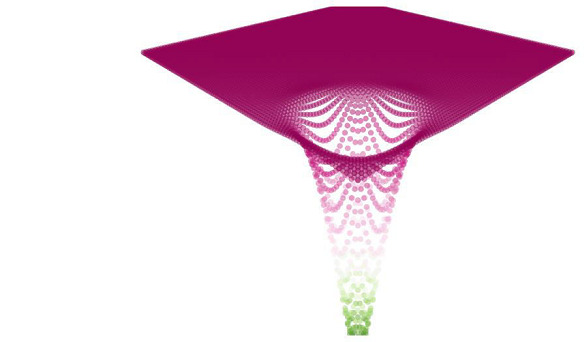# Implementation of Teaching Learning Based Optimization

## Introduction

Teaching Learning Based Optimization (TLBO) is based on the relationship between a teacher and the learners in a class. In a particular class, a teacher imparts knowledge to the students through his/her hard work. The students or learners then interact with each other among themselves and improve their knowledge.

## What is TLBO?

Let us consider a population p (particularly a class) and the number of learners l in the class. There may be decisive variables (subjects from which learners gain knowledge) for the optimization problem. Two modes of learning can happen−

• Through the teacher (Teaching phase)

• Through the interaction of the learners among themselves (Learning phase)

We are concerned about the result for the learner which will be the fitness value.

## TLBO optimization functions

There are two types of functions involved in the optimization algorithm. They are

• The Sphere function − For evaluating the performance

It is written mathematically as,

$$\mathrm{f(x_{1,}x_{2},.........x_{n})=\sum ^{n}_{i=0} \:\:x^{2}_{i}}$$

min at f(0,..0) = 0

• The Rastrigin function − Non-convex function used as a test function It is given mathematically as,

$$\mathrm{f(x_{1,}x_{2},.........x_{n})=10+}\mathrm{\sum_{i=1}^{n} (x^{2}_{i}-10\cos\:\:\cos(2\prod x_{i})}$$

## Implementation of TLBO Algorithm in Python

### Example

import numpy as np
from pyMetaheuristic.algorithm import teaching_learning_based_optimization
from pyMetaheuristic.utils import graphs

def eas_opt(varval = [0, 0]):
x_1, x_2 = varval
fval = -np.cos(x_1) * np.cos(x_2) * np.exp(-(x_1 - np.pi) ** 2 - (x_2 - np.pi) ** 2)
return fval
plt_params = {
'min_values': (-6, -6),
'max_values': (6, 6),
'step': (0.2, 0.2),
'solution': [],
'proj_view': '3D',
'view': 'notebook'
}
graphs.plot_single_function(target_function = eas_opt, **plt_params)

params = {
'population_size': 15,
'min_values': (-5, -5),
'max_values': (5, 5),
'generations': 500,
'verbose': True
}
tlbo = teaching_learning_based_optimization(target_function = eas_opt, **params)

vars = tlbo[:-1]
min = tlbo[ -1]
print('Variables: ', np.around(vars, 5) , ' Minimum Value Found: ', round(min, 5) )

plt_params = {
'min_values': (-6, -6),
'max_values': (6, 6),
'step': (0.2, 0.2),
'solution': [vars],
'proj_view': '3D',
'view': 'notebook'
}
graphs.plot_single_function(target_function = eas_opt, **plt_params)


### OutputGeneration = 0 f(x) = -0.5748727344288006
Generation = 1 f(x) = -0.7555913129284719
Generation = 2 f(x) = -0.9219320357862593
Generation = 3 f(x) = -0.9644524112155972
Generation = 4 f(x) = -0.9809361915349301
Generation = 5 f(x) = -0.991863434885587
Generation = 6 f(x) = -0.9984949247685845
Generation = 7 f(x) = -0.9991563851570532
Generation = 8 f(x) = -0.9997584334443873
Generation = 9 f(x) = -0.9997584334443873
Generation = 10 f(x) = -0.9998450580252695
Generation = 11 f(x) = -0.9998982502404465
Generation = 12 f(x) = -0.999961847330126
Generation = 13 f(x) = -0.9999810734164969
Generation = 14 f(x) = -0.9999930426674921
Generation = 15 f(x) = -0.9999995055655798
Generation = 16 f(x) = -0.9999999410594664
Generation = 17 f(x) = -0.9999999410594664
Generation = 18 f(x) = -0.9999999877205884
Generation = 19 f(x) = -0.9999999877205884
Generation = 20 f(x) = -0.9999999931826284
-------------------------------------------------------------
-------------------------------------------------------
Generation = 500 f(x) = -0.9999999991966855
Variables: [3.14161 3.14158] Minimum Value Found: -1.0


## Advantages of Teaching Learning-Based Optimization

• TLBO algorithm does not require any parameter for its working except two parameters which are the size of the population and iteration count.

• It is more accurate and it does not need derivative,

• Follows the complete path to generate the solution

## Disadvantages of Teaching Learning-Based Optimization

• It is a time-consuming method

• A huge amount of space is required for the Optimization algorithm to run

## Conclusion

Teaching Learning Based Optimization is a population-based algorithm that heavily relies on the learning relationship between teacher and learners and also among learners themselves.